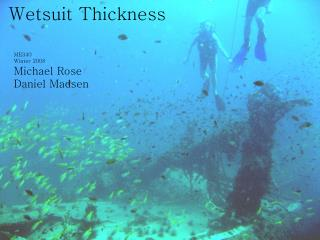DownloadDownload PresentationWetsuit Thickness

# Wetsuit Thickness

Download Presentation## Wetsuit Thickness

- - - - - - - - - - - - - - - - - - - - - - - - - - - E N D - - - - - - - - - - - - - - - - - - - - - - - - - - -
##### Presentation Transcript

1. Wetsuit Thickness ME340 Winter 2008 Michael Rose Daniel Madsen

2. Project Objective • To create a program which calculates the thickness for custom neoprene wetsuits that will maintain an individual at a comfortable body temperature.

3. Physical Model H • The human body is modeled as a cylinder with an internal core surrounded by: • a 3 mm layer of skin • a 1 mm layer of water • a wetsuit of unknown thickness • forced convection currents from surrounding water Dependent on water temp. C = Diver’s waist size

4. Thermal Analysis Body Core Temperature Outside Water Temperature Skin Forced Convection Water Layer Wetsuit q • Constant heat generation from body depending on weight: Where Tc= 98.6 degrees Fahrenheit, and:

5. Thermal Analysis cont. • Calculating the convective coefficient: • Velocities estimated at a maximum of 3-4 m/s due to swimming speed of diver. • Nusselt number equation used for flat plate in parallel flow. (Outer surface of cylinder modeled as flat plate). • Wetsuit thickness (t)is calculated from the above equations by the program using Newton-Rhapson numerical method.

6. User Interface How does it all work? The program uses a series of algorithms to perform the necessary calculations. First, the input values are converted into metric values. The heat generation is calculated using a linear curve fit of data based on calories burned during scuba diving. The properties of water are determined based on the water temperature input by the user. This is done through a database lookup. Interpolation is used as needed. The forced convection of the water is determined using the properties of water at the given temperature. Finally, the Newton-Rhapson method is used to solve for the wetsuit thickness. • User inputs: • Height (H) [in.] • Waist Size (C) [in.] • Weight (W) [lbs] • Water Temperature • Outputs: • Wetsuit Thickness (t) [mm] • Screen Shot of the User-Interface